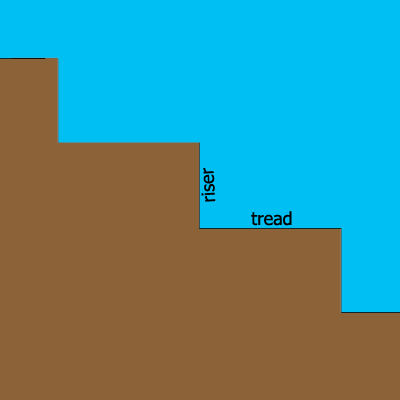SEARCH HOMEMath Central Quandaries & QueriesQuestion from Emily, a student: Continuing tia's math question about rise to run. Plans for a set of stairs for the front of a new community center use the ratio of rise to run of 2 units to 5 units. B. Sketch a set of stairs that meets the rise-to-run ratio of 2 units to 5 units. That's all, and thank you for taking the time to help me!Hi Emily,

The problem doesn't tell you the number of steps. You will need to decide that yourself. I drew a couple of steps to illustrate.Plans for stairs use the terms "tread" and "riser" as illustrated in the diagram. Mathematicians call the length of the tread the "run" and the length of the riser the "rise". In my diagram the run is 5 units and the rise is 3 units. Decide on how many steps you need and draw a diagram to scale where the rise is 2 units and the run is 5 units.

PennyMath Central is supported by the University of Regina and The Pacific Institute for the Mathematical Sciences.